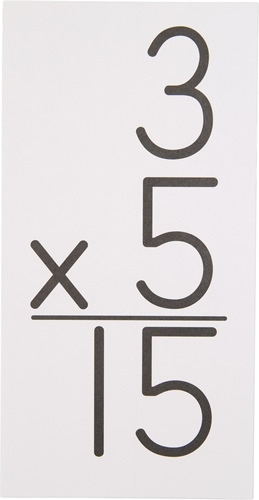•  My Math ClassHow To Help Your Student: 4th Grade Math is based mostly on multiplication facts.  Please ensure your child is practicing his or her flash cards regularly.  Fourth Grade focuses on double digit multiplication, long division, fractions, using common and uncommon denominators for addition and subtraction.  All of these rely on knowing basic math facts.In my math class, students are expected to solve each problem assigned by doing the following:1. Write the problem2. Show how they solve it (strategy)3. Write the answer.All classwork, quizzes, tests and homework are expected to be completed this way. I teach strategies for solving problems, and by showing their work, students are preparing for higher grades, learning where they made their mistakes, and demonstrating comprehension of the various strategies. In my math class we have the following routine: Daily: In class we learn new concepts every day.  Students keep a vocabulary section of their notebooks  for every new math vocabulary term we study.  With each new concept they copy an example from the board they can use to complete their homework. Homework is given out in class and started during class.  If students do not finish it needs to be done that night. Math 4 Today is designed to reinforce skills taught in 3rd grade for student to keep their basic math calculations fresh.   Math 4 Today is a worksheet given out every Monday and one row is completed every night as homework. Each row consists of four 3rd grade level review math problems. On Fridays: students are given a Math 4 Today (M4T) Quiz which follows the pattern of the questions reviewed during the week.  The quiz is based on concepts learned in 3rd grade.  Careful attention to detail and solving each problem using strategies we learn in class are key.